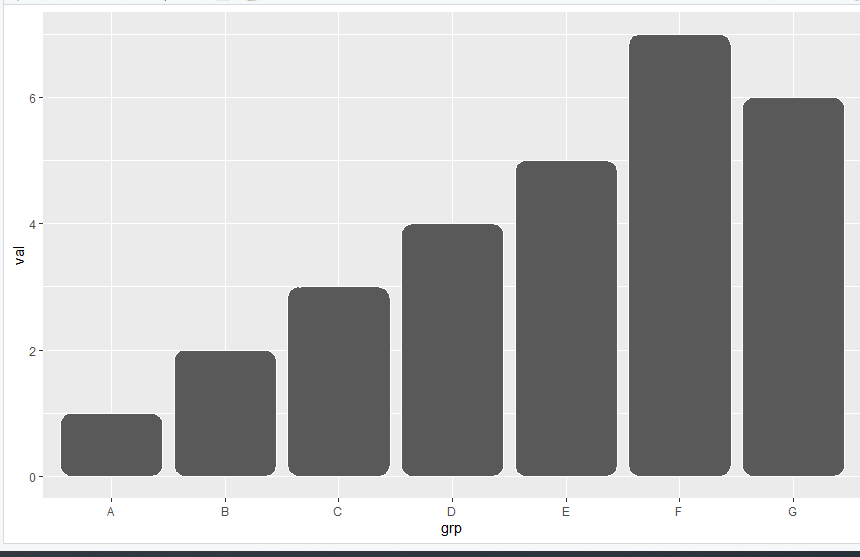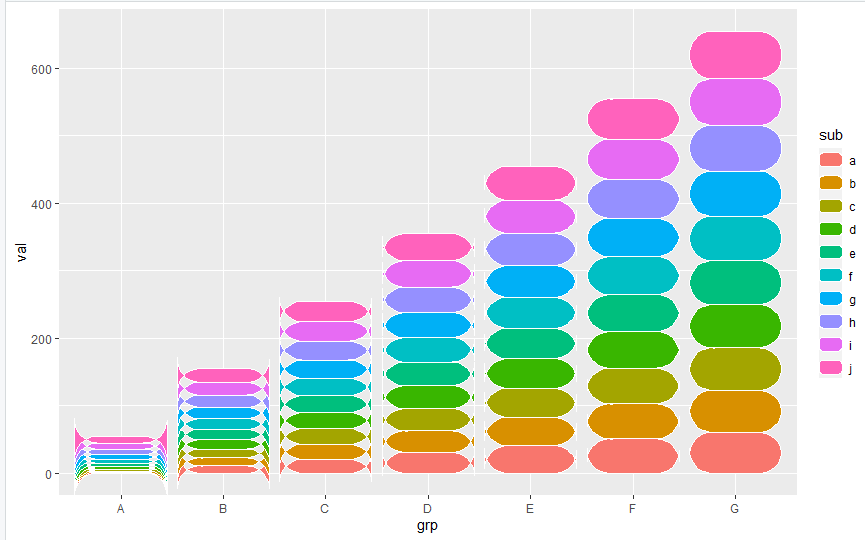GFG App
Open AppBrowser
Continue

# Draw ggplot2 Barplot With Round Corners in R

In this article, we will be looking at the various approaches to drawing ggplot2 barplot with round corners in the R programming language.

## Method 1: Draw ggplot2 Barplot with Round Corners Using ggchicklet Package

In this approach to drawing a ggplot2 barplot with round corners, the user needs to firstly install and import the ggchicklet package to the working console, then call the geom_chicklet() function of the ggchicklet package with the ggplot() function of the ggplot2 package passed with the required parameters accordingly to both the functions and this will be resulting to the barplot with the round corners in the R programming language.

Syntax to install and import the ggchicklet package:

install.packages(“ggchicklet”,repos = “https://cinc.rud.is”)

library(“ggchicklet”)

Example:

In this example, we have created data of 7points with 7groups and then with the use of the ggplot we have created a simple bar chart, further, for the curve, we have called the greom_chicklet() function with the required parameters in the R programming language.

## R

 `# Import required libraries` `library``(``"ggplot2"``)` `library``(``"ggchicklet"``)`   `# Create example data` `data < - ``data.frame``(val=``c``(1, 2, 3, 4, 5, 7, 6),` `                    ``grp=``c``(``'A'``, ``'B'``, ``'C'``, ``'D'``,` `                          ``'E'``, ``'F'``, ``'G'``))`   `# ggplot2 barplot with round corners` `ggplot``(data, ``aes``(grp, val)) +` `geom_chicklet``(radius = grid:: ``unit``(3, ``"mm"``))`

Output:## Method 2: Draw Stacked ggplot2 Barplot with Round Corners Using ggchicklet Package

In this approach to drawing a stacked ggplot2 barplot with the round corners, here also the user firstly needs to install and import the ggchicklet and the ggplot2 package to use if the functionality to the working console, and further the user needs to

Example:

In this example, we have created data of 70 points with 7 groups with 10 subgroups and then with the use of the ggplot we have created a stacked bar chart, further, for the curve, we have called the greom_chicklet() function with the required parameters and the diameter to the curve set to 6 mm in the R programming language.

## R

 `# Import required libraries` `library``(``"ggplot2"``)` `library``(``"ggchicklet"``)`   `# Create example data` `data < -   ``data.frame``(val=1: 70,` `                      ``grp=``rep``(``LETTERS``[1:7], each=10),` `                      ``sub=``letters``[1:10])`   `# ggplot2 barplot with round corners` `ggplot``(data, ``aes``(grp, val, fill=sub)) +` `geom_chicklet``(radius = grid:: ``unit``(6, ``"mm"``))`

Output:My Personal Notes arrow_drop_up